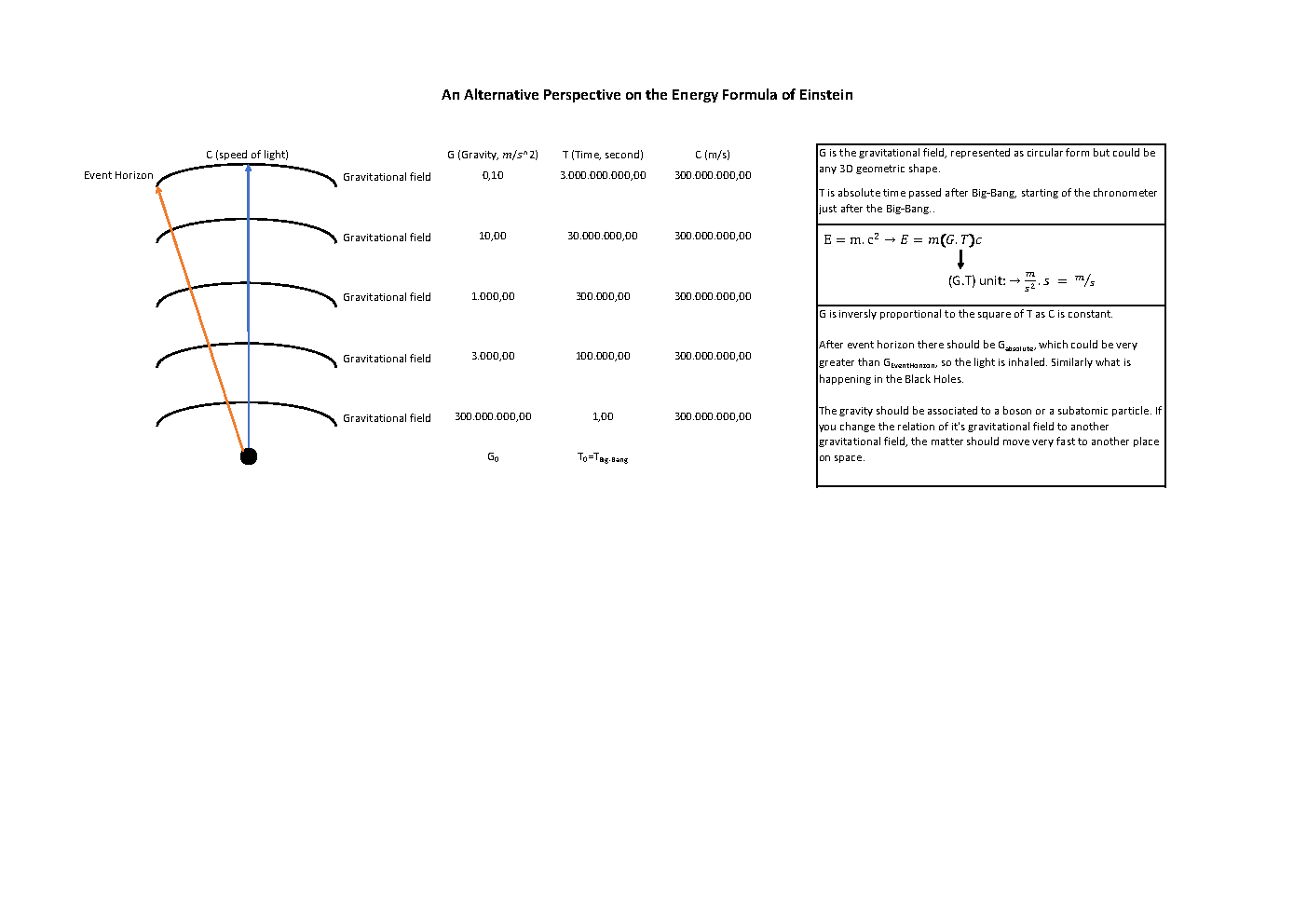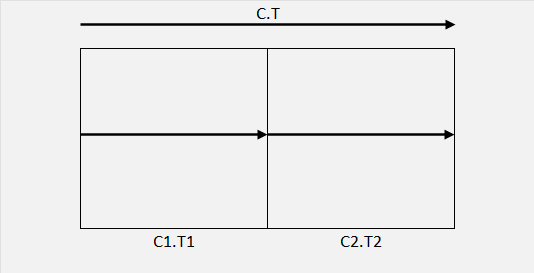# Alternative approach on Einstein's Energy Formula

#### lyingbuddha

Hello All,

I hope this is the right place to send this thread. What do you think about below formulation? The numbers are added as sample only to represent the logic.

I have added as an image but you can also find the PDF version here: PDF file with better resolution.#### Catastrophe

##### "There never was a good war, or a bad peace."
Can you explain what you are getting at please?

Cat#### lyingbuddha

Hello again. I'm still on the way to get to a point.

What I'm trying to say is, speed of light should be equal to gravity * time. So the equation should be: C=G.T

As C is constant, if gravity increases, time value decreases. For example in a black hole, the gravity is huge so the time value should be very small. At the event horizon of a black hole, the time value will be equal to zero, so we observe the light as steady, "at the same time" it is inhaled by the black hole's singularity.

Mathematically, where T is zero, G value could be (+ - infinite), So we can assume gravitational field existed before Big Bang. All universe was filled with a vast gravitational field. Then, Big Bang happened, time started to tick on, light started to travel in the space, particles occurred and started to stick on the gravitational fields (which should be continuously being generated as still time elapses).

I consider time value as "time elapsed after Big-Bang", but this is maybe more complicated than counting seconds with a chronometer. When big bang happened there was a huge gravity in space, so the seconds should be passing slower than as of today on Earth. Vice versa, if we go to the outer parts of the space, where the gravity is less, time should be ticking faster.

So if we put gravity and time factor into the energy formula, E=m(G.T)C.

#### Catastrophe

##### "There never was a good war, or a bad peace."
Isn't it easier if you just put everything moving at the speed of light through spacetime?

Cat#### lyingbuddha

If you are observing from origin of Big Bang, yes.#### Catastrophe

##### "There never was a good war, or a bad peace."
Just to clarify, I meant at the speed of light through spacetime, not through space.

Cat#### lyingbuddha

Well, after your emphasis I have searched about the spacetime over google, it looks very complex to me. Can't tell anything about it.Nevertheless, I assume there should be a universal time which we can calculate by T=C/G.

We know at the moment of Big Bang there was a huge gravity so basically the time (universal time) should be very close to zero. Before that, there was no light so the speed of light was zero, so the universal time was zero. Our journey starts with the light, isn't it? Well maybe we can observe earlier than that? If there is a ray (some sort of infrared light) that can travel faster than light (basically a light type that is less effected by gravity), we can observe if the gravity existed before Big Bang (and of course after the Event Horizon).

#### Catastrophe

##### "There never was a good war, or a bad peace."

The speed of light is a universal constant. It keeps appearing.
According to well informed authors,
"Evenly if you are perfectly stationary , the spacetime distance
you travel = ct. You are moving in spacetime at the speed of light.
Everything moves in spacetime at the speed of light."

In my opinion, this could limit our perception within the time cone to produce the illusion of time. Time is just an illusion due to the ct element in the equation.

Cat#### lyingbuddha

I understand like this:
Let's say everything is perfectly stationary and all space is traveling C.T in spacetime.

Let's assume the space is divided into 2 equal parts: 2 different speed of light (C1, C2) traveling left to right, in time elapsed T=T1+T2.Total distance traveled is C.T=C1.T1+C2.T2
The speed of light equation is C=C1+C2
Time elapsed equation is T=T1+T2
So if we put C.T to the left side of the equation:
(C1+C2)(T1+T2) = C1.T1+C2.T2
→ C1.T1+C1.T2+C2.T1+C2.T2 = C1.T1+C2.T2
C1.T1+C1.T2+C2.T1+C2.T2 = C1.T1+C2.T2
→ C1.T2+C2.T1 = 0

If we say time elapsed can not be less than zero, this equation can be true in 3 conditions below:
(I) C1=0 & T1=0 (first part of the space does not exist)
(II) C2=0 & T2=0 (second part of the space does not exist)
(III) We divided space into two equal parts so we can consider T1=T2 so → C1.T2 = - C2.T1

If there is a speed of light vector in positive direction, there should be a speed of light vector in negative direction.

Future and past cones are something similar to this?

#### Catastrophe

##### "There never was a good war, or a bad peace."
The speed of light is the same for all observers, so C1 = C2 = C = C1 + C2, and T1 = T2.

So the whole construct breaks down, doesn't it?

It is better if you work in 4-dimensions, x, y, z, and t.
For light beam from origin going to x, y, z, in time t,

x^2 + y^2 + z^2 - (ct)^2 = 0 and take it from there.

Cat#### lyingbuddha

I will check this out, thanks for directing.

I'm still thinking on the C=G.T equation. I think the order of the formation of the planets are related to their gravity.

If you check the table below, the formation order could be like this.

 ORDER C (m/s) G (m/s2) T (s) 1 Sun 299.792.458,00 274,00 1.094.133,06 2 Jupiter 299.792.458,00 23,10 12.978.028,48 3 Neptune 299.792.458,00 11,00 27.253.859,82 4 Earth 299.792.458,00 9,80 30.591.067,14 5 Saturn 299.792.458,00 9,00 33.310.273,11 6 Venus 299.792.458,00 8,90 33.684.545,84 7 Uranus 299.792.458,00 8,70 34.458.903,22 8 Mercury 299.792.458,00 3,70 81.024.988,65 8 Mars 299.792.458,00 3,70 81.024.988,65 9 Moon 299.792.458,00 1,60 187.370.286,25 10 Pluto 299.792.458,00 0,70 428.274.940,00 11 Ceres 299.792.458,00 0,30 999.308.193,33

The solar system:After the Sun's formation, first Jupiter appears in the middle with a huge gravity, then Neptune at the far outside with lesser but big gravity, then Earth from inner side, then Saturn from outside then Venus from inside, after that Uranus from outside then Mercury and Mars from inside (maybe at the same time). Then the rest of the dwarf planets and satellites like moon with small gravity. I feel like there is a pattern of gravitational fields on this order. I consider T is universal time elapsed between the creation of these gravitational fields.

#### Catastrophe

##### "There never was a good war, or a bad peace."
I think a sequential process is inevitable. But with the different mechanisms in/out of the frost line, different processes would be taking place at the same time. Don;t forget the material the planets are made of is gradually being blown away of the Solar Wind.

With the other question, start with a 4-d representation of (spacetime) with the coordinates x, y, z, and t (time). To have compatible coordinates the time coordinate is ct (km/sec)/sec = km or length. Some major names agree with this. I will add these later.

Imagine the lightning strike as from the origin (our centre) to the place with coordinates x, y, z, and t. The length of this line is ct (km/sec)(sec) = length.
If you come back to the origin, this is the centre of our terms of reference. Each person has their own centre of reference (relativity), so if you put everyone at their origin (x, y, z = 0) then they are all travelling at c through spacetime (to get from origin to point T - end of lightning strike).

#### lyingbuddha

Well, light and time creates a lot of problem, so I'd like to remove these two from C=G.T equation.

C=λ.f → f=C/λ,
T=1/f → C=G/f,
→ C=G.λ/C → C^2=G.λ,
E=m.C^2 → E=m.G.λ (unit is kg * m/s^2 * m = kg.m^2/s^2)

I have calculated both (E1) E=mc^2 and (E2) E=m.G.λ.
E1 is bigger than E2 so the lambda should be around 10^16 in order to get the E2 result to a comparable margin to E1.
Of course there should be another corrections, I am just making an approximate calculation.
So, I have checked the ratio between E1/E2, the result is below. The order is not related to mass (since both formulas has this factor) but gravity. What do you think about this?

 E1 E2 ORDER Spatial C (m/s) G (m/s2) T (s) Freq (1/s) Mass (kg) λ (m) E=m.c^2 E=m.G.λ E1/E2 Ratio 1 Sun 299.792.458,00 274,00 1.094.133,06 9,13966E-07 1,988E+30 1E+16 1,78673E+47 5,44712E+48 0,03 2 Jupiter 299.792.458,00 23,10 12.978.028,48 7,70533E-08 1,898E+27 1E+16 1,70584E+44 4,38438E+44 0,39 3 Neptune 299.792.458,00 11,00 27.253.859,82 3,66921E-08 1,02E+26 1E+16 9,1673E+42 1,122E+43 0,82 4 Earth 299.792.458,00 9,80 30.591.067,14 3,26893E-08 5,97E+24 1E+16 5,36557E+41 5,8506E+41 0,92 5 Saturn 299.792.458,00 9,00 33.310.273,11 3,00208E-08 5,68E+26 1E+16 5,10493E+43 5,112E+43 1,00 6 Venus 299.792.458,00 8,90 33.684.545,84 2,96872E-08 4,87E+24 1E+16 4,37694E+41 4,3343E+41 1,01 7 Uranus 299.792.458,00 8,70 34.458.903,22 2,90201E-08 8,68E+25 1E+16 7,80119E+42 7,5516E+42 1,03 8 Mars 299.792.458,00 3,70 81.024.988,65 1,23419E-08 6,42E+23 1E+16 5,77001E+40 2,3754E+40 2,43 8 Mercury 299.792.458,00 3,70 81.024.988,65 1,23419E-08 3,3E+23 1E+16 2,96589E+40 1,221E+40 2,43 9 Moon 299.792.458,00 1,60 187.370.286,25 5,33703E-09 7,3E+22 1E+16 6,56091E+39 1,168E+39 5,62 10 Pluto 299.792.458,00 0,70 428.274.940,00 2,33495E-09 1,3E+22 1E+16 1,16838E+39 9,1E+37 12,84 11 Ceres 299.792.458,00 0,30 999.308.193,33 1,00069E-09 9,1E+20 1E+16 8,17867E+37 2,73E+36 29,96